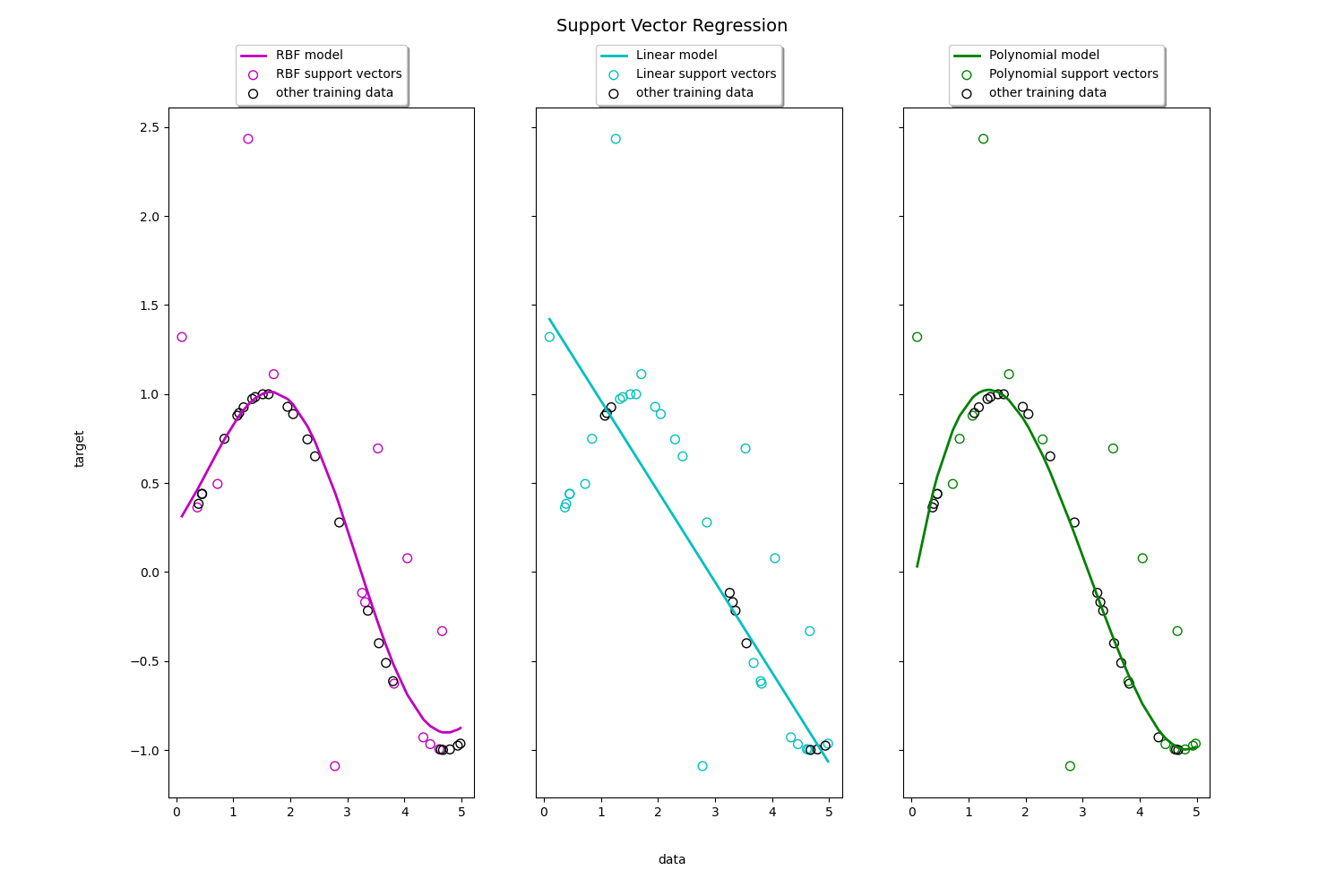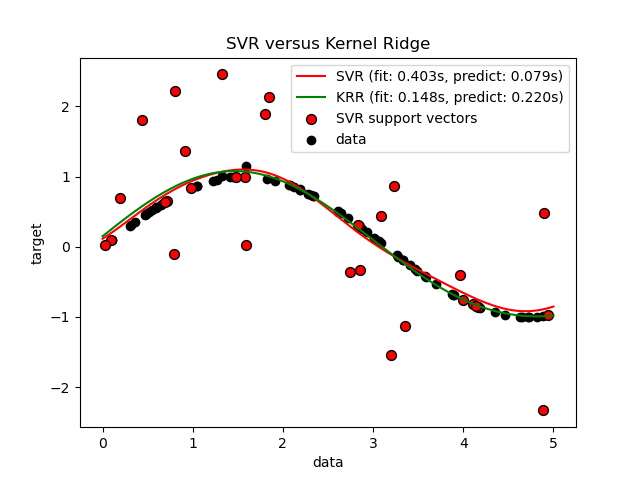# sklearn.svm.SVR¶

class sklearn.svm.SVR(*, kernel='rbf', degree=3, gamma='scale', coef0=0.0, tol=0.001, C=1.0, epsilon=0.1, shrinking=True, cache_size=200, verbose=False, max_iter=-1)

Epsilon支持向量回归。

kernel {‘linear’, ‘poly’, ‘rbf’, ‘sigmoid’, ‘precomputed’}, 默认=’rbf’

degree 整数型，默认=3

gamma 浮点数或者{‘scale’, ‘auto’} , 默认=’scale’

coef0 浮点数，默认=0.0

tol 浮点数，默认=1e-3

C 浮点数，默认= 1.0

epsilon 浮点数, 默认= 0.1
epsilon-SVR模型中的Epsilon。它指定了在训练损失函数中预测值与实际值之间距离为epsilon的epsilon-tube。
shrinking 布尔值，默认=True

cache_size 浮点数，默认=200

verbose 布尔值，默认=False

max_iter 整数型，默认=-1

support_ 形如(n_SV,)的数组

support_vectors_ 形如(n_SV, n_features)的数组

n_support_ 形如(n_class)的数组，dtype=int32

dual_coef_ 形如(n_class-1, n_SV)的数组

coef_ 形如(n_class * (n_class-1) / 2, n_features)的数组 分配给特征的权重（原始问题的系数），仅在线性内核的情况下可用。coef_是一个继承自raw_coef_的只读属性，它遵循liblinear的内部存储器布局。
fit_status_ 整数型

intercept_ 形如(n_class * (n_class-1) / 2,)的数组

NuSVR

LinearSVC

>>> from sklearn.svm import SVR>>> from sklearn.pipeline import make_pipeline>>> from sklearn.preprocessing import StandardScaler>>> import numpy as np>>> n_samples, n_features = 10, 5>>> rng = np.random.RandomState(0)>>> y = rng.randn(n_samples)>>> X = rng.randn(n_samples, n_features)>>> regr = make_pipeline(StandardScaler(), SVR(C=1.0, epsilon=0.2))>>> regr.fit(X, y)Pipeline(steps=[('standardscaler', StandardScaler()),                ('svr', SVR(epsilon=0.2))])

fit(X, y[, sample_weight]) 根据给定的训练数据拟合支持向量机模型。
get_params([deep]) 获取这个估计器的参数。
predict(X) 在X中对样本进行分类
score(X, y[, sample_weight]) 返回预测的决定系数R^2。
set_params(**params) 设置这个估计器的参数。
__init__(*, kernel='rbf', degree=3, gamma='scale', coef0=0.0, tol=0.001, C=1.0, epsilon=0.1, shrinking=True, cache_size=200, verbose=False, max_iter=-1)

fit(X, y, sample_weight=None)

X 形如(n_samples, n_features) 或者 (n_samples, n_samples)的数组或者稀疏矩阵

y 形如(n_samples,)的数组

sample_weight 形如(n_samples,)的数组，默认=None

self object

get_params(deep=True)

deep bool, default = True

params mapping of string to any

predict(X)

X 形如(n_samples, n_features) 或者 (n_samples_test, n_samples_train)的数组或者稀疏矩阵

y_pred 形如(n_sample, )的数组
X中样本的类别标签。
score(X, y, sample_weight=None)

X 形如 (n_samples, n_features)的数组

y 形如(n_samples,) 或者 (n_samples, n_outputs)的数组
X中结果为真的标签
sample_weight 形如(n_samples,)的数组，默认=None

score 浮点数
self.predict(X) wrt. y的R^2

set_params(**params)

params 字典

self object

## sklearn.svm.SVR使用示例¶